Publicité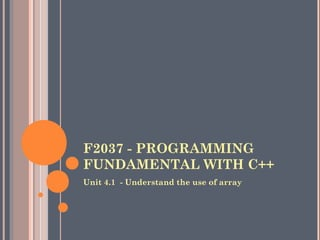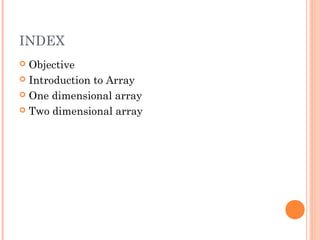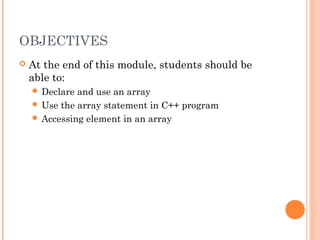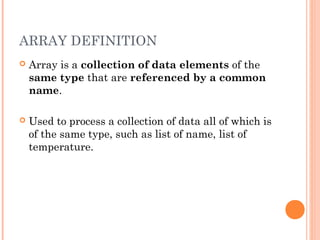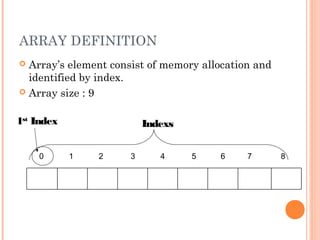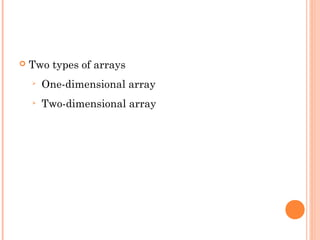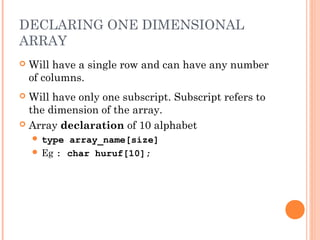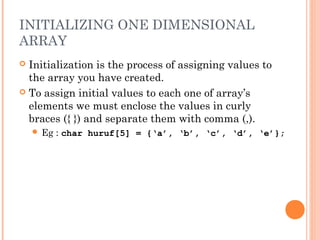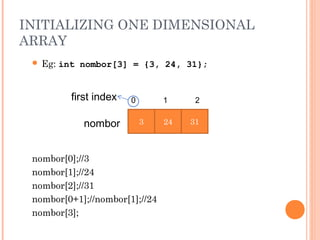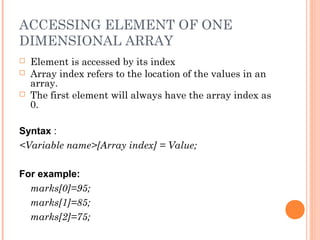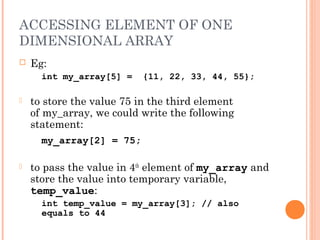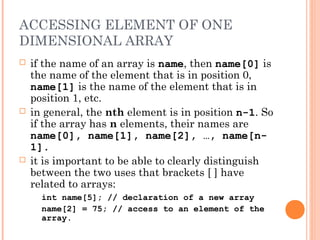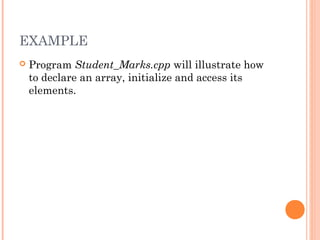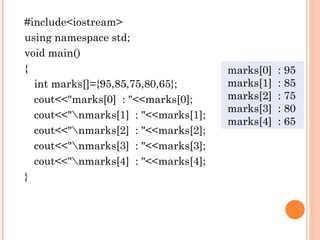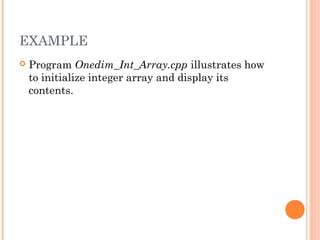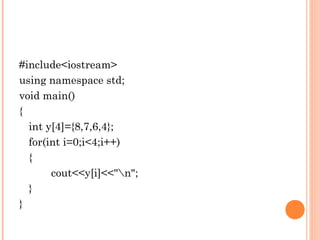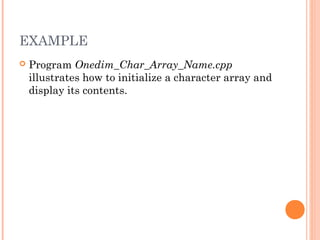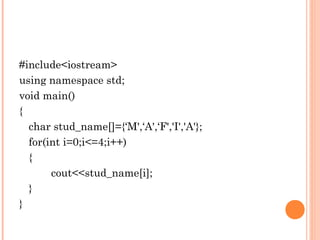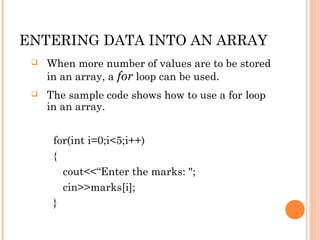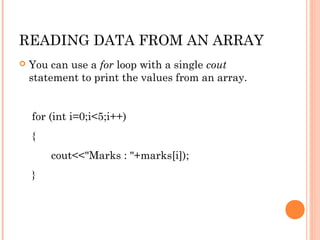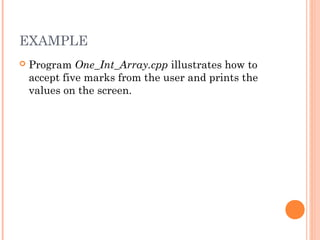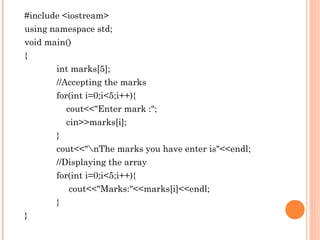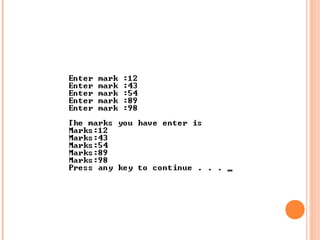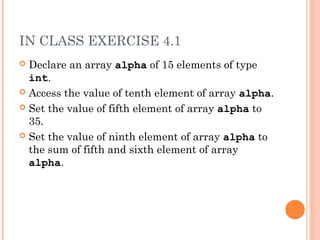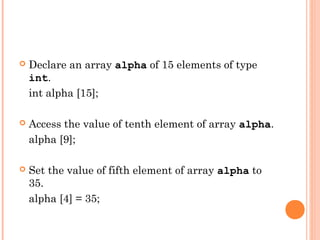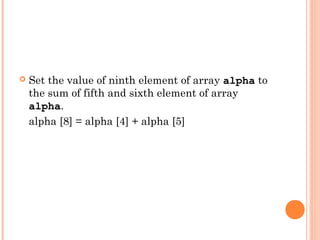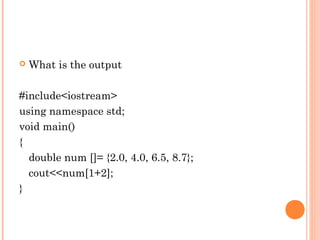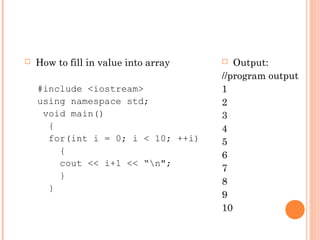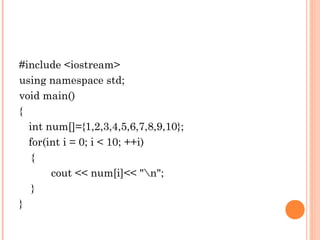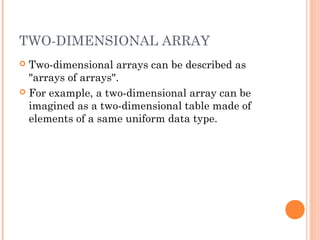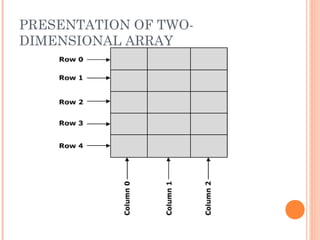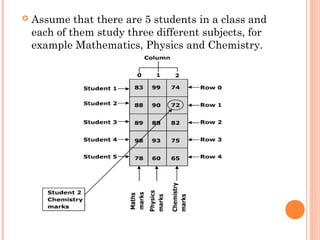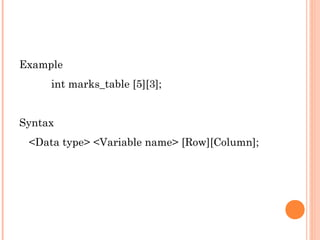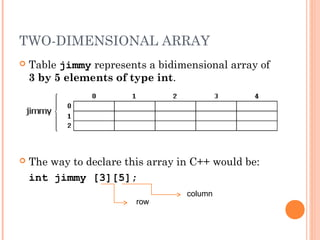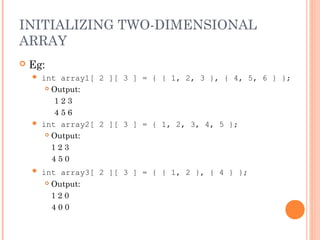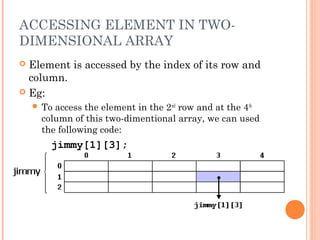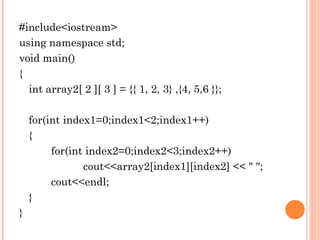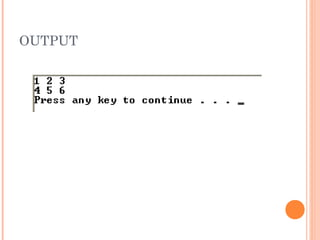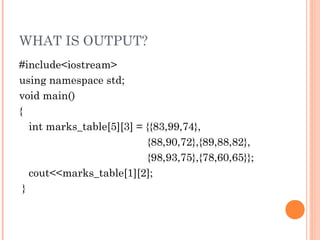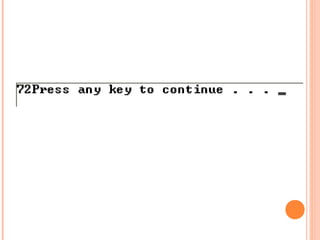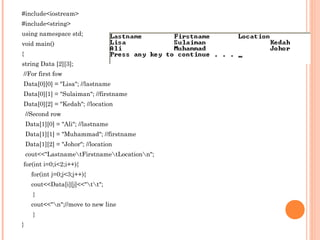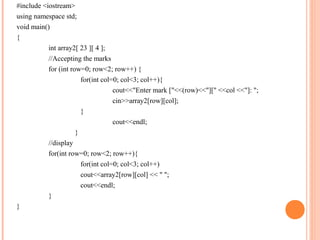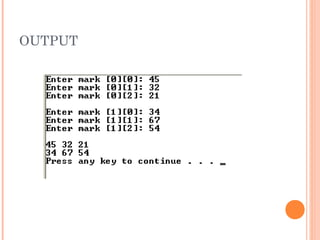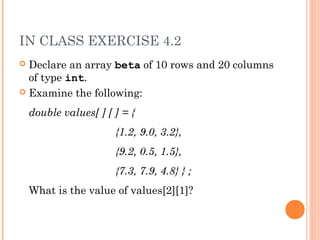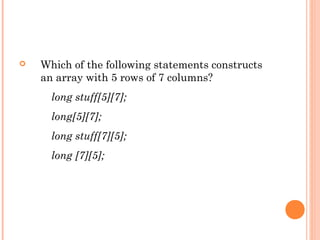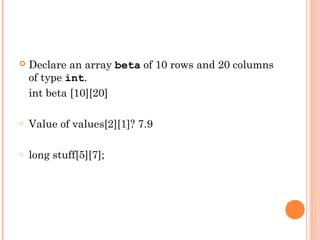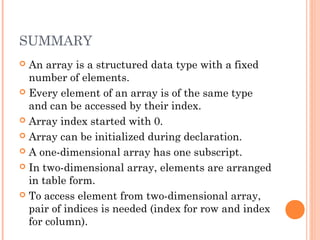1 sur 47
Publicité

### Fp201 unit4

1. F2037 - PROGRAMMING FUNDAMENTAL WITH C++ Unit 4.1 - Understand the use of array
2. INDEX  Objective  Introduction to Array  One dimensional array  Two dimensional array
3. OBJECTIVES  At the end of this module, students should be able to:  Declare and use an array  Use the array statement in C++ program  Accessing element in an array
4. ARRAY DEFINITION  Array is a collection of data elements of the same type that are referenced by a common name.  Used to process a collection of data all of which is of the same type, such as list of name, list of temperature.
5. ARRAY DEFINITION  Array’s element consist of memory allocation and identified by index.  Array size : 9 1st Index Indexs 0 1 2 3 4 5 6 7 8
6. Two types of arrays  One-dimensional array  Two-dimensional array
7. DECLARING ONE DIMENSIONAL ARRAY  Will have a single row and can have any number of columns.  Will have only one subscript. Subscript refers to the dimension of the array.  Array declaration of 10 alphabet  type array_name[size]  Eg : char huruf;
8. INITIALIZING ONE DIMENSIONAL ARRAY  Initialization is the process of assigning values to the array you have created.  To assign initial values to each one of array’s elements we must enclose the values in curly braces ({ }) and separate them with comma (,).   Eg : char huruf = {‘a’, ‘b’, ‘c’, ‘d’, ‘e’};
9. INITIALIZING ONE DIMENSIONAL ARRAY  Eg: int nombor = {3, 24, 31}; first index 0 1 2 nombor 3 24 31 nombor;//3 nombor;//24 nombor;//31 nombor[0+1];//nombor;//24 nombor;
10. ACCESSING ELEMENT OF ONE DIMENSIONAL ARRAY  Element is accessed by its index  Array index refers to the location of the values in an array.  The first element will always have the array index as 0. Syntax : <Variable name>[Array index] = Value; For example: marks=95; marks=85; marks=75;
11. ACCESSING ELEMENT OF ONE DIMENSIONAL ARRAY  Eg: int my_array = {11, 22, 33, 44, 55};  to store the value 75 in the third element of my_array, we could write the following statement: my_array = 75;  to pass the value in 4th element of my_array and store the value into temporary variable, temp_value: int temp_value = my_array; // also equals to 44
12. ACCESSING ELEMENT OF ONE DIMENSIONAL ARRAY  if the name of an array is name, then name is the name of the element that is in position 0, name is the name of the element that is in position 1, etc.  in general, the nth element is in position n-1. So if the array has n elements, their names are name, name, name, …, name[n- 1].  it is important to be able to clearly distinguish between the two uses that brackets [ ] have related to arrays: int name; // declaration of a new array name = 75; // access to an element of the array.
13. EXAMPLE  Program Student_Marks.cpp will illustrate how to declare an array, initialize and access its elements.
14. #include<iostream> using namespace std; void main() { marks : 95 int marks[]={95,85,75,80,65}; marks : 85 cout<<"marks : "<<marks; marks : 75 marks : 80 cout<<"nmarks : "<<marks; marks : 65 cout<<"nmarks : "<<marks; cout<<"nmarks : "<<marks; cout<<"nmarks : "<<marks; }
15. EXAMPLE  Program Onedim_Int_Array.cpp illustrates how to initialize integer array and display its contents.
16. #include<iostream> using namespace std; void main() { int y={8,7,6,4}; for(int i=0;i<4;i++) { cout<<y[i]<<"n"; } }
17. EXAMPLE  Program Onedim_Char_Array_Name.cpp illustrates how to initialize a character array and display its contents.
18. #include<iostream> using namespace std; void main() { char stud_name[]={‘M',‘A',‘F','I','A'}; for(int i=0;i<=4;i++) { cout<<stud_name[i]; } }
19. ENTERING DATA INTO AN ARRAY  When more number of values are to be stored in an array, a for loop can be used.  The sample code shows how to use a for loop in an array. for(int i=0;i<5;i++) { cout<<“Enter the marks: "; cin>>marks[i]; }
20. READING DATA FROM AN ARRAY  You can use a for loop with a single cout statement to print the values from an array. for (int i=0;i<5;i++) { cout<<"Marks : "+marks[i]); }
21. EXAMPLE  Program One_Int_Array.cpp illustrates how to accept five marks from the user and prints the values on the screen.
22. #include <iostream> using namespace std; void main() { int marks; //Accepting the marks for(int i=0;i<5;i++){ cout<<"Enter mark :"; cin>>marks[i]; } cout<<"nThe marks you have enter is"<<endl; //Displaying the array for(int i=0;i<5;i++){ cout<<"Marks:"<<marks[i]<<endl; } }
23. IN CLASS EXERCISE 4.1  Declare an array alpha of 15 elements of type int.  Access the value of tenth element of array alpha.  Set the value of fifth element of array alpha to 35.  Set the value of ninth element of array alpha to the sum of fifth and sixth element of array alpha.
24. Declare an array alpha of 15 elements of type int. int alpha ;  Access the value of tenth element of array alpha. alpha ;  Set the value of fifth element of array alpha to 35. alpha  = 35;
25. Set the value of ninth element of array alpha to the sum of fifth and sixth element of array alpha. alpha  = alpha  + alpha 
26. What is the output #include<iostream> using namespace std; void main() { double num []= {2.0, 4.0, 6.5, 8.7}; cout<<num[1+2]; }
27. How to fill in value into array  Output: //program output #include <iostream> 1 using namespace std; 2 void main() 3 { 4 for(int i = 0; i < 10; ++i) 5 { 6 cout << i+1 << “n"; 7 } 8 } 9 10
28. #include <iostream> using namespace std; void main() { int num[]={1,2,3,4,5,6,7,8,9,10}; for(int i = 0; i < 10; ++i) { cout << num[i]<< "n"; } }
29. TWO-DIMENSIONAL ARRAY  Two-dimensional arrays can be described as "arrays of arrays".  For example, a two-dimensional array can be imagined as a two-dimensional table made of elements of a same uniform data type.
30. PRESENTATION OF TWO- DIMENSIONAL ARRAY
31. Assume that there are 5 students in a class and each of them study three different subjects, for example Mathematics, Physics and Chemistry.
32. Example int marks_table ;   Syntax <Data type> <Variable name> [Row][Column];
33. TWO-DIMENSIONAL ARRAY  Table jimmy represents a bidimensional array of 3 by 5 elements of type int.  The way to declare this array in C++ would be: int jimmy ; column row
34. INITIALIZING TWO-DIMENSIONAL ARRAY  Eg:  int array1[ 2 ][ 3 ] = { { 1, 2, 3 }, { 4, 5, 6 } };  Output: 123 456  int array2[ 2 ][ 3 ] = { 1, 2, 3, 4, 5 };  Output: 123 450  int array3[ 2 ][ 3 ] = { { 1, 2 }, { 4 } };  Output: 120 400
35. ACCESSING ELEMENT IN TWO- DIMENSIONAL ARRAY  Element is accessed by the index of its row and column.  Eg:  Toaccess the element in the 2nd row and at the 4th column of this two-dimentional array, we can used the following code: jimmy;
36. #include<iostream> using namespace std; void main() { int array2[ 2 ][ 3 ] = {{ 1, 2, 3} ,{4, 5,6 }}; for(int index1=0;index1<2;index1++) { for(int index2=0;index2<3;index2++) cout<<array2[index1][index2] << " "; cout<<endl; } }
37. OUTPUT
38. WHAT IS OUTPUT? #include<iostream> using namespace std; void main() { int marks_table = {{83,99,74}, {88,90,72},{89,88,82}, {98,93,75},{78,60,65}}; cout<<marks_table; }
39. #include<iostream> #include<string> using namespace std; void main() { string Data ; //For first fow Data = "Lisa"; //lastname Data = "Sulaiman"; //firstname Data = "Kedah"; //location //Second row Data = "Ali"; //lastname Data = "Muhammad"; //firstname Data = "Johor"; //location cout<<"LastnametFirstnametLocationn"; for(int i=0;i<2;i++){ for(int j=0;j<3;j++){ cout<<Data[i][j]<<"tt"; } cout<<"n";//move to new line } }
40. #include <iostream> using namespace std; void main() { int array2[ 23 ][ 4 ]; //Accepting the marks for (int row=0; row<2; row++) { for(int col=0; col<3; col++){ cout<<"Enter mark ["<<(row)<<"][" <<col <<"]: "; cin>>array2[row][col]; } cout<<endl; } //display for(int row=0; row<2; row++){ for(int col=0; col<3; col++) cout<<array2[row][col] << " "; cout<<endl; } }
41. OUTPUT
42. IN CLASS EXERCISE 4.2  Declare an array beta of 10 rows and 20 columns of type int.  Examine the following: double values[ ] [ ] = { {1.2, 9.0, 3.2}, {9.2, 0.5, 1.5}, {7.3, 7.9, 4.8} } ; What is the value of values?
43. Which of the following statements constructs an array with 5 rows of 7 columns? long stuff; long; long stuff; long ;
44. Declare an array beta of 10 rows and 20 columns of type int. int beta  o Value of values? 7.9 o long stuff;
45. SUMMARY  An array is a structured data type with a fixed number of elements.  Every element of an array is of the same type and can be accessed by their index.  Array index started with 0.  Array can be initialized during declaration.  A one-dimensional array has one subscript.  In two-dimensional array, elements are arranged in table form.  To access element from two-dimensional array, pair of indices is needed (index for row and index for column).
Publicité